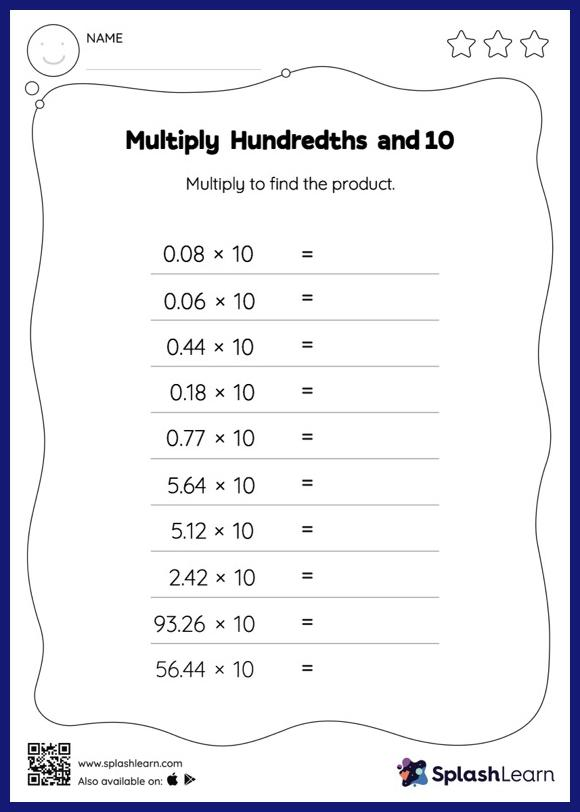# Multiply Hundredths and 10: Horizontal Multiplication Worksheet

Home > Multiply Hundredths and 10: Horizontal MultiplicationThe decimal point moves one place to the right when a decimal is multiplied by 10. Multiply hundredths and 10 worksheet requires students to apply this concept. As the worksheet uses a horizontal format, it allows for more creativity when it comes to how the student solves the problem. In contrast, in the vertical format, students usually employ the standard method to solve.